# QLineF Class

The QLineF class provides a two-dimensional vector using floating point precision. More...

 Header: #include qmake: QT += core

## Public Types

 (alias) IntersectionType

## Public Functions

 QLineF(const QLine &line) QLineF(qreal x1, qreal y1, qreal x2, qreal y2) QLineF(const QPointF &p1, const QPointF &p2) QLineF() QPointF p1() const QPointF p2() const qreal x1() const qreal x2() const qreal y1() const qreal y2() const qreal angle() const qreal angleTo(const QLineF &line) const QPointF center() const qreal dx() const qreal dy() const QLineF::IntersectionType intersects(const QLineF &line, QPointF *intersectionPoint) const bool isNull() const qreal length() const QLineF normalVector() const QPointF pointAt(qreal t) const void setP1(const QPointF &p1) void setP2(const QPointF &p2) void setAngle(qreal angle) void setLength(qreal length) void setLine(qreal x1, qreal y1, qreal x2, qreal y2) void setPoints(const QPointF &p1, const QPointF &p2) QLine toLine() const void translate(const QPointF &offset) void translate(qreal dx, qreal dy) QLineF translated(const QPointF &offset) const QLineF translated(qreal dx, qreal dy) const QLineF unitVector() const bool operator!=(const QLineF &line) const bool operator==(const QLineF &line) const

## Static Public Members

 QLineF fromPolar(qreal length, qreal angle)
 QDataStream & operator<<(QDataStream &stream, const QLineF &line) QDataStream & operator>>(QDataStream &stream, QLineF &line)

## Detailed Description

A QLineF describes a finite length line (or line segment) on a two-dimensional surface. QLineF defines the start and end points of the line using floating point accuracy for coordinates. Use the toLine() function to retrieve an integer based copy of this line.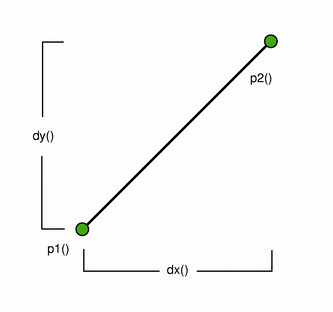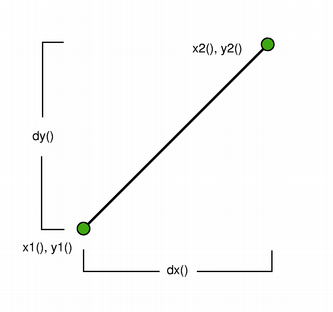The positions of the line's start and end points can be retrieved using the p1(), x1(), y1(), p2(), x2(), and y2() functions. The dx() and dy() functions return the horizontal and vertical components of the line, respectively.

The line's length can be retrieved using the length() function, and altered using the setLength() function. Similarly, angle() and setAngle() are respectively used for retrieving and altering the angle of the line. Use the isNull() function to determine whether the QLineF represents a valid line or a null line.

The intersects() function determines the IntersectionType for this line and a given line, while the angleTo() function returns the angle between the lines. In addition, the unitVector() function returns a line that has the same starting point as this line, but with a length of only 1, while the normalVector() function returns a line that is perpendicular to this line with the same starting point and length.

Finally, the line can be translated a given offset using the translate() function, and can be traversed using the pointAt() function.

### Constraints

QLine is limited to the minimum and maximum values for the `int` type. Operations on a QLine that could potentially result in values outside this range will result in undefined behavior.

## Member Type Documentation

### `[alias] `QLineF::IntersectionType

This is a type alias for IntersectType.

Describes the intersection between two lines.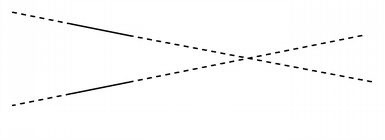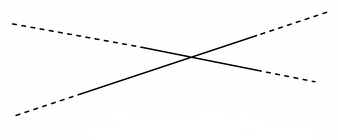QLineF::UnboundedIntersection QLineF::BoundedIntersection
ConstantDescription
`NoIntersection`Indicates that the lines do not intersect; i.e. they are parallel.
`UnboundedIntersection`The two lines intersect, but not within the range defined by their lengths. This will be the case if the lines are not parallel. intersect() will also return this value if the intersect point is within the start and end point of only one of the lines.
`BoundedIntersection`The two lines intersect with each other within the start and end points of each line.

## Member Function Documentation

### QLineF::QLineF(const QLine &line)

Construct a QLineF object from the given integer-based line.

### QLineF::QLineF(qrealx1, qrealy1, qrealx2, qrealy2)

Constructs a line object that represents the line between (x1, y1) and (x2, y2).

### QLineF::QLineF(const QPointF &p1, const QPointF &p2)

Constructs a line object that represents the line between p1 and p2.

### QLineF::QLineF()

Constructs a null line.

### QPointF QLineF::p1() const

Returns the line's start point.

### QPointF QLineF::p2() const

Returns the line's end point.

### qreal QLineF::x1() const

Returns the x-coordinate of the line's start point.

### qreal QLineF::x2() const

Returns the x-coordinate of the line's end point.

### qreal QLineF::y1() const

Returns the y-coordinate of the line's start point.

### qreal QLineF::y2() const

Returns the y-coordinate of the line's end point.

### qreal QLineF::angle() const

Returns the angle of the line in degrees.

The return value will be in the range of values from 0.0 up to but not including 360.0. The angles are measured counter-clockwise from a point on the x-axis to the right of the origin (x > 0).

This function was introduced in Qt 4.4.

### qreal QLineF::angleTo(const QLineF &line) const

Returns the angle (in degrees) from this line to the given line, taking the direction of the lines into account. If the lines do not intersect within their range, it is the intersection point of the extended lines that serves as origin (see QLineF::UnboundedIntersection).

The returned value represents the number of degrees you need to add to this line to make it have the same angle as the given line, going counter-clockwise.

This function was introduced in Qt 4.4.

### QPointF QLineF::center() const

Returns the center point of this line. This is equivalent to 0.5 * p1() + 0.5 * p2().

This function was introduced in Qt 5.8.

### qreal QLineF::dx() const

Returns the horizontal component of the line's vector.

### qreal QLineF::dy() const

Returns the vertical component of the line's vector.

### `[static] `QLineF QLineF::fromPolar(qreallength, qrealangle)

Returns a QLineF with the given length and angle.

The first point of the line will be on the origin.

Positive values for the angles mean counter-clockwise while negative values mean the clockwise direction. Zero degrees is at the 3 o'clock position.

This function was introduced in Qt 4.4.

### QLineF::IntersectionType QLineF::intersects(const QLineF &line, QPointF *intersectionPoint) const

Returns a value indicating whether or not this line intersects with the given line.

The actual intersection point is extracted to intersectionPoint (if the pointer is valid). If the lines are parallel, the intersection point is undefined.

This function was introduced in Qt 5.14.

### bool QLineF::isNull() const

Returns `true` if the line does not have distinct start and end points; otherwise returns `false`. The start and end points are considered distinct if qFuzzyCompare() can distinguish them in at least one coordinate.

Note: Due to the use of fuzzy comparison, isNull() may return `true` for lines whose length() is not zero.

### qreal QLineF::length() const

Returns the length of the line.

### QLineF QLineF::normalVector() const

Returns a line that is perpendicular to this line with the same starting point and length.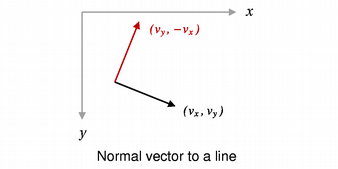### QPointF QLineF::pointAt(qrealt) const

Returns the point at the parameterized position specified by t. The function returns the line's start point if t = 0, and its end point if t = 1.

### void QLineF::setP1(const QPointF &p1)

Sets the starting point of this line to p1.

This function was introduced in Qt 4.4.

### void QLineF::setP2(const QPointF &p2)

Sets the end point of this line to p2.

This function was introduced in Qt 4.4.

### void QLineF::setAngle(qrealangle)

Sets the angle of the line to the given angle (in degrees). This will change the position of the second point of the line such that the line has the given angle.

Positive values for the angles mean counter-clockwise while negative values mean the clockwise direction. Zero degrees is at the 3 o'clock position.

This function was introduced in Qt 4.4.

### void QLineF::setLength(qreallength)

Sets the length of the line to the given length. QLineF will move the end point - p2() - of the line to give the line its new length, unless length() was previously zero, in which case no scaling is attempted. For lines with very short lengths (represented by denormal floating-point values), results may be imprecise.

### void QLineF::setLine(qrealx1, qrealy1, qrealx2, qrealy2)

Sets this line to the start in x1, y1 and end in x2, y2.

This function was introduced in Qt 4.4.

### void QLineF::setPoints(const QPointF &p1, const QPointF &p2)

Sets the start point of this line to p1 and the end point of this line to p2.

This function was introduced in Qt 4.4.

### QLine QLineF::toLine() const

Returns an integer based copy of this line.

Note that the returned line's start and end points are rounded to the nearest integer.

### void QLineF::translate(const QPointF &offset)

Translates this line by the given offset.

### void QLineF::translate(qrealdx, qrealdy)

Translates this line the distance specified by dx and dy.

### QLineF QLineF::translated(const QPointF &offset) const

Returns this line translated by the given offset.

This function was introduced in Qt 4.4.

### QLineF QLineF::translated(qrealdx, qrealdy) const

Returns this line translated the distance specified by dx and dy.

This function was introduced in Qt 4.4.

### QLineF QLineF::unitVector() const

Returns the unit vector for this line, i.e a line starting at the same point as this line with a length of 1.0, provided the line is non-null.

### bool QLineF::operator!=(const QLineF &line) const

Returns `true` if the given line is not the same as this line.

A line is different from another line if their start or end points differ, or the internal order of the points is different.

### bool QLineF::operator==(const QLineF &line) const

Returns `true` if the given line is the same as this line.

A line is identical to another line if the start and end points are identical, and the internal order of the points is the same.

## Related Non-Members

### QDataStream &operator<<(QDataStream &stream, const QLineF &line)

Writes the given line to the given stream and returns a reference to the stream.